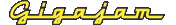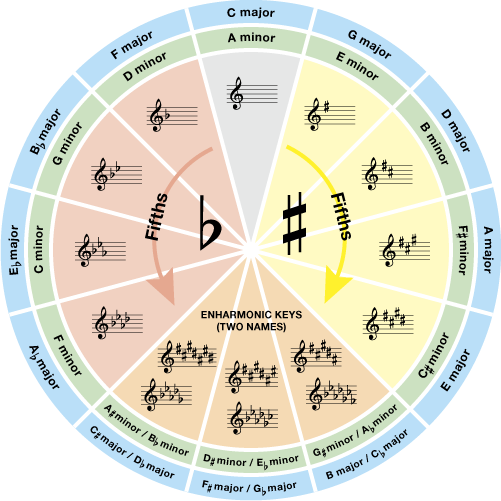EMT Lesson Four  How to understand keys and key signatures

# Circle of fifths

The cycle of fifths is most commonly demonstrated in the ‘circle of fifths’ as shown in Figure 20.

Figure 20. The circle of fifths.   (Enlarge)Figure 20. The circle of fifths. (PDF 75KB)

Next: Relative minor keys